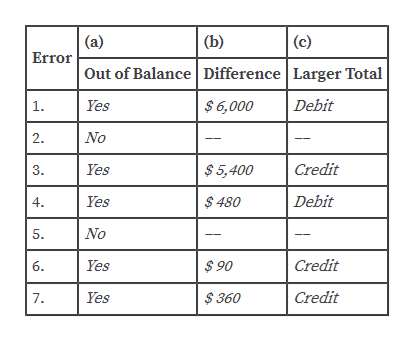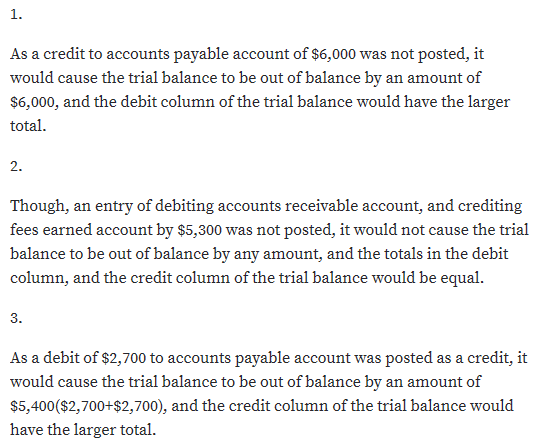# The following errors occurred in posting from a two-column journal:1. A credit of \$6,000 to Accounts Payable was not posted.2. An entry debiting Accounts Receivable and crediting Fees Earned for \$5,300 was not posted.3. A debit of \$2,700 to Accounts Payable was posted as a credit.4. A debit of \$480 to Supplies was posted twice.5. A debit of \$3,600 to Cash was posted to Miscellaneous Expense.6. A credit of \$780 to Cash was posted as \$870.7. A debit of \$12,620 to Wages Expense was posted as \$12,260.Considering each case individually (i.e., assuming that no other errors had occurred), indicate (a) by “yes” or “no” whether the trial balance would be out of balance; (b) if answer to (a) is “yes,” the amount by which the trial balance totals would differ; and (c) whether the Debit or Credit column of the trial balance would have the larger total. Answers should be presented in the following form, with error (1) given as an example:                (a)                        (b)                                       (c)Error    Out of Balance      Difference Larger                Total1.            yes                       \$6,000                                 debit

Question
6 views

The following errors occurred in posting from a two-column journal:
1. A credit of \$6,000 to Accounts Payable was not posted.
2. An entry debiting Accounts Receivable and crediting Fees Earned for \$5,300 was not posted.
3. A debit of \$2,700 to Accounts Payable was posted as a credit.
4. A debit of \$480 to Supplies was posted twice.
5. A debit of \$3,600 to Cash was posted to Miscellaneous Expense.
6. A credit of \$780 to Cash was posted as \$870.
7. A debit of \$12,620 to Wages Expense was posted as \$12,260.
Considering each case individually (i.e., assuming that no other errors had occurred), indicate (a) by “yes” or “no” whether the trial balance would be out of balance; (b) if answer to (a) is “yes,” the amount by which the trial balance totals would differ; and (c) whether the Debit or Credit column of the trial balance would have the larger total. Answers should be presented in the following form, with error (1) given as an example:
(a)                        (b)                                       (c)
Error    Out of Balance      Difference Larger                Total
1.            yes                       \$6,000                                 debit

check_circle

Step 1

The error that would cause the trial balance to be out of balance, the amount by which the trial balance totals would differ, and the debit or credit columns of the trial balance totals would differ, and the debit or credit column of the trial balance having the larger totals are as follows:help_outlineImage Transcriptionclose(a) Error (b) (c) Out of Balance Difference Larger Total Debit Yes \$6,000 1. No 2. Credit Yes \$5,400 3. Debit Yes \$480 4. No 5. Credit Yes \$90 6. Credit Yes \$360 7. fullscreen
Step 2...

### Want to see the full answer?

See Solution

#### Want to see this answer and more?

Solutions are written by subject experts who are available 24/7. Questions are typically answered within 1 hour.*

See Solution
*Response times may vary by subject and question.
Tagged in